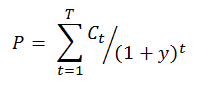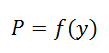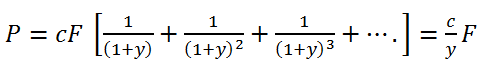# Price Yield Relationship for Bonds

For a bond with a cash flow pattern the present value can be calculated using the following formula:Where the numerator denotes the coupon or principal in that period, t is the number of periods, T is the number of periods to final maturity and y is the discount factor per period.

For a bond the cash flows involved are the fixed coupon payment and the repayment of principal or face value at expiration.

There are three cases:

• When the coupon rate matches the yield the price of the bond will equal to its face value and it is referred to in the market as a par bond.
• The case where the coupon is lesser then the yield results in a price lesser than the face value and this is termed as a discount bond.
• When the coupon is greater than the yield the price will exceed the face value and the bond becomes a premium bond.

The above equation indicates the relationship between the price and yield of the bond given its structure.  The price of the bond is a non-linear function of the yield y and can be represented by the following equation –If a transaction happens between coupon payments then the price of the bond termed as the dirty price of the bond needs to include the coupon interest that has been accrued between the last coupon payment and the transaction date. The yield that is computed in this case is the implied yield. The price computed without the accrued interest is called the clean price.

The relationship between the price and the yield of the bond is highly non-linear. When yield is is zero the price of the bond is simply the sum of the cash flows the bond. If the coupon is equal to the yield then the price is the same as the face value and when the yield tends to very large values the price tends to zero. This is called the payoff function and for yields very close to the coupon the relationship is quasi-linear.

In case where the bond makes regular coupon payments without a maturity date it is called a consol or perpetual bond or perpetuity. The maturity of a consol is infinite and the cash flows are equal to a fixed percentage of the face value.

The formula the indicates the price of the consol is as follows –As this equation indicates, the price is inversely proportional to yield.

# R Programming Bundle: 25% OFF

Get our R Programming - Data Science for Finance Bundle for just $29$39.
Get it now for just \$29